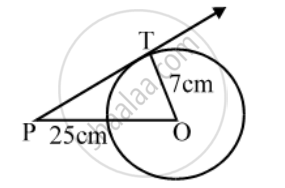# Find the length of the tangent drawn from a point whose distance from the centre of a circle is 25 cm. Given that the radius of the circle is 7 cm. - Mathematics

Sum

Find the length of the tangent drawn from a point whose distance from the centre of a circle is 25 cm. Given that the radius of the circle is 7 cm.

#### Solution

Let P be the given point, O be the centre of the circle and PT be the length of tangent from P. Then, OP = 25 cm and OT = 7 cm.Since tangent to a circle is always perpendicular to the radius through the point of contact.

∴ ∠OTP = 90°
In right triangle OTP, we have

OP^2 = OT^2 + PT^2

⇒ 25^2 = 7^2 + PT^2

⇒ PT^2 = 25^2 – 7^2

= (25 – 7) (25 + 7)

= 576

⇒ PT = 24 cm

Hence, length of tangent from P = 24 cm

Concept: Concept of Circle - Centre, Radius, Diameter, Arc, Sector, Chord, Segment, Semicircle, Circumference, Interior and Exterior, Concentric Circles
Is there an error in this question or solution?
Share